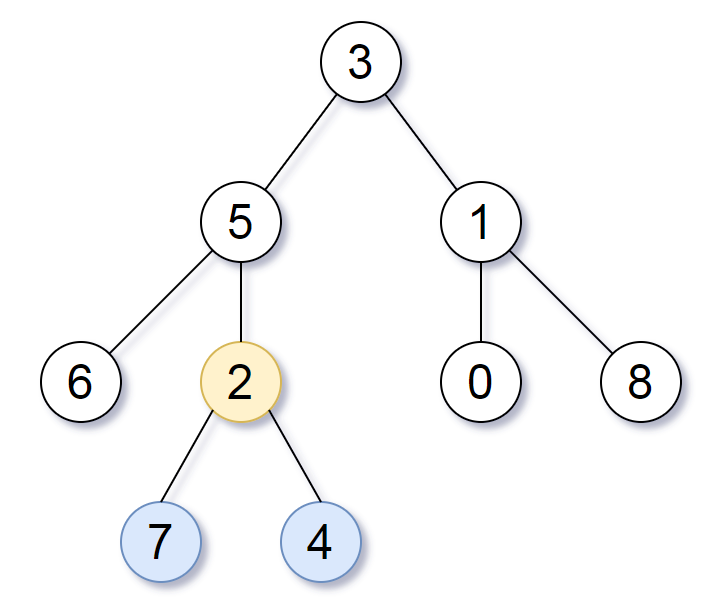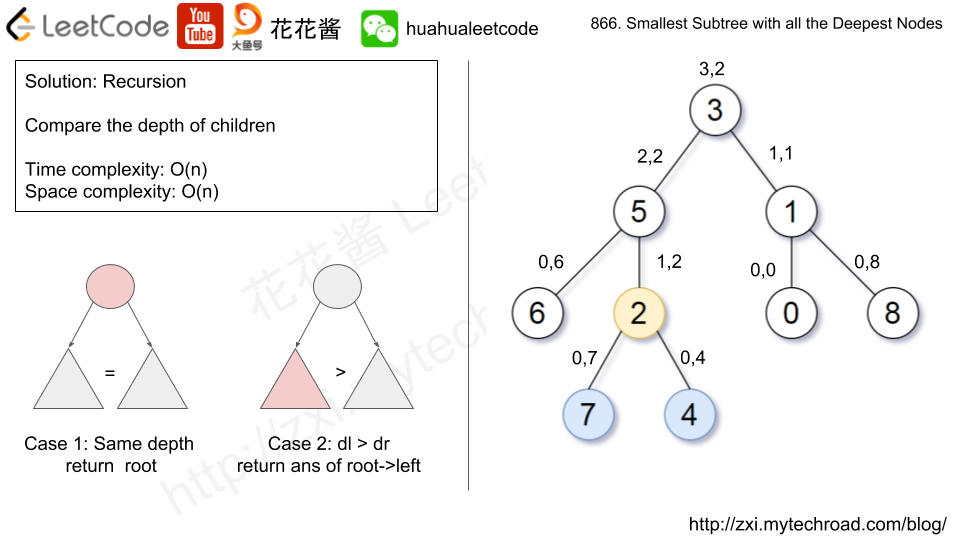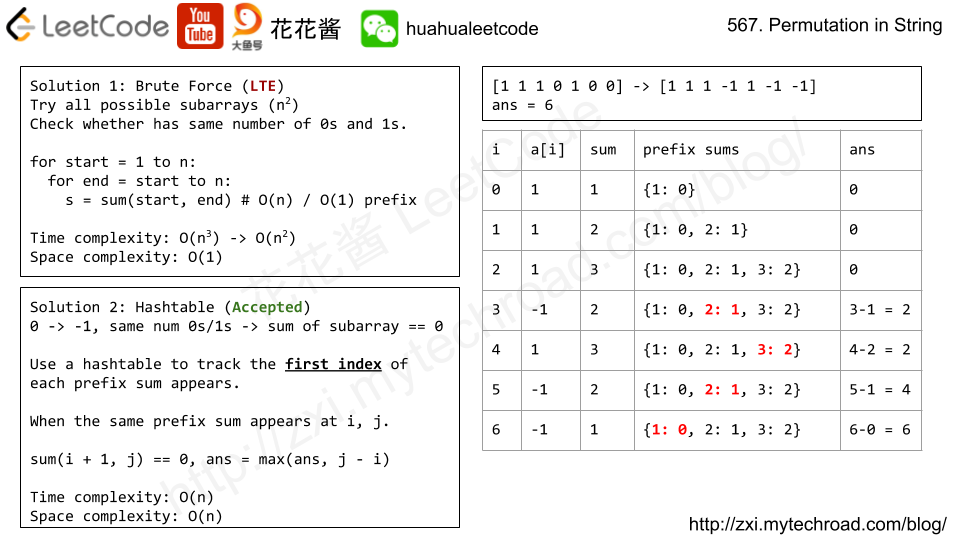# Problem

Given the root node of a binary search tree (BST) and a value to be inserted into the tree, insert the value into the BST. Return the root node of the BST after the insertion. It is guaranteed that the new value does not exist in the original BST.

Note that there may exist multiple valid ways for the insertion, as long as the tree remains a BST after insertion. You can return any of them.

For example,

You can return this binary search tree:

This tree is also valid:

# Solution: Recursion

Time complexity: O(logn ~ n)

Space complexity: O(logn ~ n)

# Problem

We have some permutation A of [0, 1, ..., N - 1], where N is the length of A.

The number of (global) inversions is the number of i < j with 0 <= i < j < N and A[i] > A[j].

The number of local inversions is the number of i with 0 <= i < N and A[i] > A[i+1].

Return true if and only if the number of global inversions is equal to the number of local inversions.

Example 1:

Input: A = [1,0,2]
Output: true
Explanation: There is 1 global inversion, and 1 local inversion.


Example 2:

Input: A = [1,2,0]
Output: false
Explanation: There are 2 global inversions, and 1 local inversion.


Note:

• A will be a permutation of [0, 1, ..., A.length - 1].
• A will have length in range [1, 5000].
• The time limit for this problem has been reduced.

# Solution1: Brute Force (TLE)

Time complexity: O(n^2)

Space complexity: O(1)

C++

# Solution2: MergeSort

Time complexity: O(nlogn)

Space complexity: O(n)

C#

# Solution3: Input Property

Input is a permutation of [0, 1, …, N – 1]

Time Complexity: O(n)

Space Complexity: O(1)

# Problem

Given a binary tree rooted at root, the depth of each node is the shortest distance to the root.

A node is deepest if it has the largest depth possible among any node in the entire tree.

The subtree of a node is that node, plus the set of all descendants of that node.

Return the node with the largest depth such that it contains all the deepest nodes in it’s subtree.

Example 1:

Input: [3,5,1,6,2,0,8,null,null,7,4]
Output: [2,7,4]
Explanation:We return the node with value 2, colored in yellow in the diagram.
The nodes colored in blue are the deepest nodes of the tree.
The input "[3, 5, 1, 6, 2, 0, 8, null, null, 7, 4]" is a serialization of the given tree.
The output "[2, 7, 4]" is a serialization of the subtree rooted at the node with value 2.
Both the input and output have TreeNode type.


Note:

• The number of nodes in the tree will be between 1 and 500.
• The values of each node are unique.# Solution: Recursion

Time complexity: O(n)

Space complexity: O(n)

C++

v2

Python3

# Problem

Given a binary array, find the maximum length of a contiguous subarray with equal number of 0 and 1.

Example 1:

Input: [0,1]
Output: 2
Explanation: [0, 1] is the longest contiguous subarray with equal number of 0 and 1.


Example 2:

Input: [0,1,0]
Output: 2
Explanation: [0, 1] (or [1, 0]) is a longest contiguous subarray with equal number of 0 and 1.


Note: The length of the given binary array will not exceed 50,000.# Solution: HashTable

Prefix sum + hashtable

Time complexity: O(n)

Space complexity: O(n)

V2: Using array instead of a hashtable

Time complexity: O(2*n + 1 + n)

Space complexity: O(2*n + 1)

# Problem

Given a sorted integer array without duplicates, return the summary of its ranges.

Example 1:

Input:  [0,1,2,4,5,7]
Output: ["0->2","4->5","7"]
Explanation: 0,1,2 form a continuous range; 4,5 form a continuous range.


Example 2:

Input:  [0,2,3,4,6,8,9]
Output: ["0","2->4","6","8->9"]
Explanation: 2,3,4 form a continuous range; 8,9 form a continuous range.

# Solution

Time complexity: O(n)

Space complexity: O(k)

C++

Mission News Theme by Compete Themes.